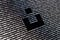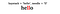# Static HTML form — how can I get data?

I was asked to build a simple HTML contact form, and building a form is an easy task. There are plenty of templates available and I thought, ok I can do it.

We fill out so many different forms online every day, but we rarely think about how it works. As a developer I needed to think about data, how would data from the form get to the site owner.

Usually, the form would store data in the database and then the site owner can access and manipulate stored data. In this case, there is no website, and HTML form…

# Time to start building again …

The time has come to get my own portfolio website

I’ve been waiting for a long time to start building my portfolio site. And even though it has been long overdue, I’ve finally started working on it.

To make it look impressive, I got help from a professional and asked my wife to get me few templates. She’s being doing website templates for a very long time and she did some great templates for me. The first part was really easy because it was done by someone else.

I decided that I will use React.js and was thinking if I…

# Question

Example 1:

`Input: root = [1,null,2,3]Output: [1,3,2]`

Example 2:

`Input: root = []Output: []`

Example 3:

`Input: root = Output: `

Example 4:

# Question

Example 1:

`Input: head = [1,1,2]Output: [1,2]`

Example 2:

# LeetCode Algorithm Challenge: Add BinaryPhoto by Alexander Sinn on Unsplash

Question

Given two binary strings `a` and `b`, return their sum as a binary string.

Example 1:

`Input: a = "11", b = "1"Output: "100"`

Example 2:

`Input: a = "1010", b = "1011"Output: "10101"`

## Let’s break it down

My first thought was “Oh it should be easy since I just did Plus One challenge, but the main difference here was the fact that we are using binary numbers.

Binary number is a number expressed in the base-2 numeral system or binary numeral system, a method of mathematical expression which uses only two symbols: typically “0” (zero) and “1” (one).

And therefore…

# Question

Given a non-empty array of decimal digits representing non-negative integers, increment the final integer by 1.

The digits are stored such that the most significant digit is at the head of the list, and each element in the array contains a single digit.

You may assume the integer does not contain any leading zero, except the number 0 itself.

Example 1:

`Input: digits = [1,2,3]Output: [1,2,4]Explanation: The array represents the integer 123.`

Example 2:

`Input: digits = [4,3,2,1]Output: [4,3,2,2]Explanation: The array represents the integer 4321.`

Example 3:

`Input: digits = Output: `

So at first…

# LeetCode Algorithm Challenge: Maximum Subarray — Brute Force versus Kadane’s Algorithm

## Question

Given an integer array ‘nums’, find the contiguous subarray (containing at least one number) which has the largest sum and return its sum.

Example 1:

Input: nums = [-2,1,-3,4,-1,2,1,-5,4]

Output: 6

Explanation: [4,-1,2,1] has the largest sum = 6.

Example 2:

Input: nums = 

Output: 1

Example 3:

Input: nums = [5,4,-1,7,8]

Output: 23

## What are we looking for?

In this task, the function should return the max sum of the subarray values. A subarray is a slice from a contiguous array (i.e., occupy consecutive positions) and inherently maintains the order of elements. …

# Question

Given a sorted array of distinct integers and a target value, return the index if the target is found. If not, return the index where it would be if it were inserted in order.

Example 1:

Input: nums = [1,3,5,6], target = 5

Output: 2

Example 2:

Input: nums = [1,3,5,6], target = 2

Output: 1

Example 3:

Input: nums = [1,3,5,6], target = 7

Output: 4

# Challenges

This task looks very simple, and at a first glance, it could be solved with the indexOf method. …

# LeetCode Algorithm Challenge: Needle in a HaystackFind the needle

# Question

Implement strStr().

Return the index of the first occurrence of needle in haystack, or -1 if needle is not part of haystack.

## Clarification

What should we return when needle is an empty string? This is a great question to ask during an interview.

For the purpose of this problem, we will return 0 when needle is an empty string. This is consistent to C’s strstr() and Java’s indexOf().

## Example 1:

Input: haystack = “hello”, needle = “ll”

Output: 2

## Example 2:

Input: haystack = “aaaaa”, needle = “bba”

Output: -1

# Challenges

1. Check if the needle exists — when the needle is an empty string return 0.

# Removing Element from an Array

This week I’ll show another LeetCode question. I find it interesting how you can solve problems by reusing pieces of code that you already have. Last week I wrote about Removing Duplicates from a Sorted Array, I will re-use parts of the code I wrote last week to solve this challenge.

# Question

“Given an array nums and a value val, remove all instances of that value in-place and return the new length. Do not allocate extra space for another array, you must do this by modifying the input array in-place with O(1) extra memory. The order of elements can be changed…